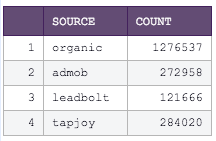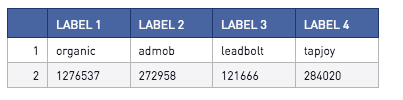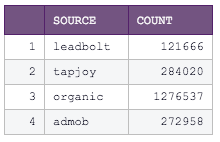Transposing Tables using Python Pandas

Pandas makes transposing tables beautifully simple! Below are a couple examples using the example SQL output below:This first Python snippet allows you to define your own column headers:

# SQL output is imported as a pandas dataframe variable called "df"
import pandas as pd

df2=df.T
df2.columns=['label 1', 'label 2','label 3','label 4']

# Use Periscope to visualize a dataframe or an image by passing data to periscope.output()
periscope.output(df2)And this Python snippet makes the first row your column headers

# SQL output is imported as a pandas dataframe variable called "df"
import pandas as pd
df2=df.T
df2=df2[1:]
# Use Periscope to visualize a dataframe or an image by passing data to periscope.output()
periscope.output(df2)Interested in learning about pivoting tables in Python or R? Check out the post here for more information.

Prefer R? Check out the community post here!

• Oldest first
• Popular•Transposing back to the original table is a little different!

Suppose your SQL output is something like this:And you wanted your output to look like this:In this case, you'd need to reset the index to preserve the original column titles as well as rename the resulting columns. The following Python code will do nicely!

# SQL output is imported as a pandas dataframe variable called "df"
import pandas as pd

df = df.T.reset_index()
df.columns = ["source", "count"]

# Use Periscope to visualize a dataframe or an image by passing data to periscope.output()
periscope.output(df)